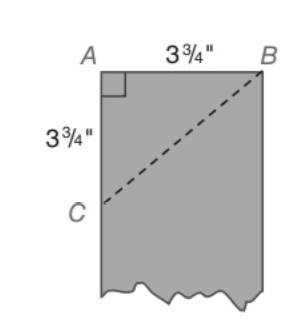Chapter 5.5, Problem 23EElementary Geometry For College St...

7th Edition
Alexander + 2 others
ISBN: 9781337614085

Solutions

Chapter
SectionElementary Geometry For College St...

7th Edition
Alexander + 2 others
ISBN: 9781337614085
Textbook Problem

A carpenter is working with a board that is 3 3 4 in. wide. After marking off a point down the side of length 3 3 4 in., the carpenter makes a cut along B C ¯ with a saw. What is the measure of the angle ( ∠ A C B ) that is formed?To determine

To find:

The measure o the angle ACB that is formed when a carpenter working with a board that is 334 in. wide. After marking off a point down the is a of length 334 in the carpenter makes a ct along BC with a saw.

Explanation

Approach:

For a right triangle for which the measure of the interior angles 45°, 45°, and 90°, if ‘a’ is the length of measure of one of the leg; opposite to the angle 45°, then the length of the other two sides is given by

Length of the other leg =a

Length of the hypotenuse =a2

In general

Length of the legs are equal.

Length of the hypotenuse =2× (Length of one of the legs)

Calculation:

Given,

A triangular piece of board that cut off from a board of wide 334 in along its diagonal that meets the opposite side of the board 334 in down the side of length

Still sussing out bartleby?

Check out a sample textbook solution.

See a sample solution

The Solution to Your Study Problems

Bartleby provides explanations to thousands of textbook problems written by our experts, many with advanced degrees!

Get Started

Convert the expressions in Exercises 6584 to power form. xy23

Finite Mathematics and Applied Calculus (MindTap Course List)

Evaluate the integral. 11t(1t)2dt

Calculus (MindTap Course List)

Demand The demand function for a product is given by where x is the number o...

Mathematical Applications for the Management, Life, and Social Sciences

Find the remaining trigonometric ratios. 30. tan = 2, 02

Single Variable Calculus: Early Transcendentals, Volume I

True or False: is a convergent series.

Study Guide for Stewart's Multivariable Calculus, 8th